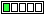All about flooble | fun stuff | Get a free chatterbox | Free JavaScript | Avatarsperplexus dot info25 balls (Posted on 2008-09-25)A bin contains 25 balls: 10 red, 8 yellow, and 7 blue. We draw three balls at random (without looking!) from the bin, and we will say that we "win" if our three balls represent exactly two colors. (That is, we "win" if we draw two balls of one color and another ball of a different color.)

What is the probability of winning in this particular game?

 See The Solution Submitted by pcbouhid No RatingComments: ( Back to comment list | You must be logged in to post comments.)solution| Comment 2 of 7 |The probability that all 3 colors would be drawn is

10/25 * 8/24 * 7/23 * 3! = 28/115

The probability that all 3 balls would be red is

10/25 * 9/24 * 8/23 = 6/115

The probability that all 3 balls would be yellow is

8/25 * 7/24 * 6/23 = 14/575

The probability that all 3 balls would be blue is

7/25 * 6/24 * 5/23 = 7/460

Then, the probability that two are of one color and one is of another is just 1 minus the total of the above. This comes out to 1529/2300 or about 0.6647826086956521738.

 Posted by Charlie on 2008-09-25 12:21:40Please log in:

 Search: Search body:
Forums (2)